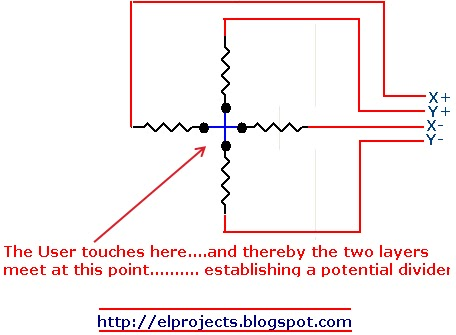# Resistive touchscreen - when measuring X, why doesn't Y affect the reading?

A resistive touchscreen (4-wire) looks like this as a schematic:To read an X position, you put voltage across X+ and X- and then read the voltage between EITHER Y pin and ground. How does the Y position of the touch not affect the reading?

Example, you put a voltage to V+ and ground V-. You press in the centre of X at the very top of the screen. So the values of East and West are equal, but North is zero and South is large. The voltmeter (or ADC in the real world) is placed across Y+ and ground, which is the same as X-. The measurement shows the voltage across East, which is what we want.

Now you press in the centre at the very bottom of the screen. South is now in series with the voltmeter, is it not? I'm still pretty green at electronic theory, but why doesn't this affect the reading? TL;DR stop reading now if that is clear, the rest is explaining because I'm not sure if it is.

To put it another way, I hope I'm not repeating myself unnecessarily, but in the below image, the measurement I WANT is assumedly the voltage across X1, but I can't get that without incorporating X4.I can't find anything to explain the effect of a resistor in series with a voltmeter, if you can point me to that it might answer my question.

Also am I correct (in this particular case) to treat an ADC as a voltmeter? The output from the ADC is over SPI, and I've got that part sorted, so I'm treating measuring the ADC output (0-1024) as the same thing as reading the voltage (0-3.3V). If I'm wrong here, this is out of scope for this question but I'd appreciate the nudge to stop me running in to a different problem.

• How would you model a voltmeter? What effect has a series resistor in that model? Similar Question – Arsenal Mar 28 '18 at 11:17

When you read X, you connect X1 and X2 across a known voltage. A press will create a potential divider at the press position. The potential divider produces a voltage proportional to the X position of the press.

That X voltage is read through a Y resistor. There will be a voltage drop across that Y resistor of, from Ohm's Law, Vry = Iry x Ry.

There will be an ADC on the other end of Y resistor Ry, ready to convert the X potential divider voltage to a binary number.

So if the current taken by the ADC input is kept very tiny then the voltage drop across Ry will also be tiny. Then the voltage that reaches the ADC will be almost exactly the same as the voltage at the X potential divider.

The actual definition of what's too small an ADC input current to disturb your X and Y resistances will depend on the resistance values in your touchscreen.

It's not difficult to make an ADC circuit with a very high input impedance, that draws practically no current from the voltage source that it's measuring. Either a suitable ADC can be used directly or the voltage can be passed through an op-amp buffer (with a gain of 1 ) with a huge input impedance.

You see a similar thing when you use your voltmeter to read the touchscreen as that has a very high input resistance.

The same principle is used for the Y measurement.

• I think I've got you. So the reading I want is Vx, and the reading I get is actually Vx minus Vry, but Vry is so small that it won't affect the real world. If I've got that right, the answer to my question is "it does, but in a negligible way." – fishkake Mar 28 '18 at 11:58